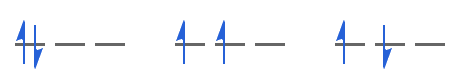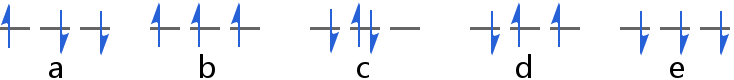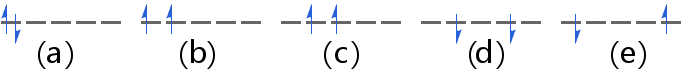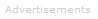# Definition of Hund's Rule

Hund's rule states that the lowest energy electron configuration, the ground state, in any electron subshell is the one with the greatest number of parallel electron spins.

Example 1
Consider the different ways in which a pair of electrons might be arranged in p orbitals. Bearing in mind that we need to satisfy the Pauli exclusion principle, the three possible ways are shown below:The middle option has the greatest number of parallel electron spins and therefore has the lowest energy, i.e. Hund's rule identifies the middle option as the electronic ground state. It is the ground state because:

• accommodating the electrons in the same orbital (the leftmost option) increases the electrostatic repulsion between the electrons because on average they are closer together
• electrons with opposite spins (the rightmost option) tend to move closer to one another than if spins are parallel (spin correlation). If electrons are closer, the electrostatic repulsion between them increases

Example 2
Here are some of the ways three electrons could be placed into p orbitals.Options (b) and (e) have the greatest number of parallel electron spins and therefore the lowest energy. Options (b) and (e) have the same energy - they are said to be degenerate orbitals.

Example 3
Here are some of the ways two electrons could be placed into d orbitals.Options (b),(c), and (d) have the greatest number of parallel electron spins and therefore the lowest energy. Options (b),(c), and (d) have the same energy - they are said to be degenerate orbitals.Search the Dictionary for More Terms